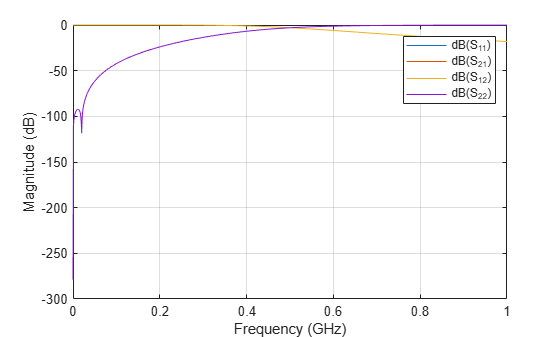## Description

`lcladder` class creates an LC ladder object that you can add to an existing circuit. Create filters and calculate S-parameters of filters using `lcladder` class. You can also add the `lcladder` object to an existing circuit.

You can also use the `lcladder` object to convert the `rffilter` object to an lcladder ```LCLad = lcladder(rffiltobj)``` where `rffiltobj` is an `rffilter` object.## Creation

### Syntax

``lcobj = lcladder(top,L,C)``
``lcobj = lcladder(rffilterobj)``
``lcobj = lcladder(___,lcname)``

### Description

example

````lcobj = lcladder(top,L,C)` creates an LC ladder object, `lcobj`, with a topology, `top`, inductor values, `L`, and capacitor values, `C`.```
````lcobj = lcladder(rffilterobj)` creates an LC ladder object, `lcobj` from an RF filter object, `rffilter`. ```
````lcobj = lcladder(___,lcname)` creates an LC ladder object, `lcobj`, with a name, `lcname`. `lcname` must be a character vector.```

## Properties

expand all

Topology type of the LC ladder, specified as a one of the following character vector:

• `'lowpasspi'`: Low-pass pi filter

• `'lowpasstee'`: Low-pass tee filter

• `'highpasspi'`: High-pass pi filter

• `'highpasstee'`: High-pass tee filter

• `'bandpasspi'`: Band-pass pi filter

• `'bandpasstee'`: Band-pass tee filter

• `'bandstoppi'`: Band-stop pi filter

• `'bandstoptee'`: Band-stop tee filter

Set the topology type in the `topology` argument of the syntax.

Example: `'lowpasspi'`

Inductor values in LC ladder, specified as a numeric scalar or vector in henries. Set the inductor value in the `inductances` argument of the syntax.

Example: `3.18e-8`

Capacitor values in LC ladder, specified as a numeric scalar or vector in farads. Set the capacitor value in the `c` argument of the syntax.

Example: `[6.37e-12 6.37e-12]`

Name of LC ladder object, specified as a character vector. Set the name of the LC ladder in `lcname` argument of the syntax.

Example: `'lcfilter'`

Number of ports in LC ladder object. specified as a scalar. This value is always `2`.

Terminal names of LC ladder object, specified as the cell vector, `{'p1+' 'p2+' 'p1-' 'p2-'}`. An LC ladder object always has four terminals: two terminals associated with the first port (`'p1+'` and `'p1-'`) and two terminals associated with the second port (`'p2+'` and `'p2-'`).

Example: `{'p1+' 'p2+' 'p1-' 'p2-'}`

Parent circuit nodes connected to LC ladder object terminals, specified as a vector of integers. This property appears only after the LC ladder object is added to a circuit.

Note

"ParentNodes" are only displayed after the capacitor has been added

into a circuit.

Full path of the circuit to which the LC ladder object belongs, specified as character vector. This path appears only after the inductor is added to the circuit.

Note

"ParentPath" is only displayed after the capacitor has been added

into a circuit.

## Object Functions

 `clone` Create copy of existing circuit element or circuit object

## Examples

collapse all

Create a low-pass pi LC ladder object with inductor value of 3.18e-8 H and capacitor value of 6.37e-12 F. Calculate and plot the s-parameters.

```L = 3.18e-8; C = [6.37e-12 6.37e-12]; lpp = lcladder('lowpasspi',L,C)```
```lpp = lcladder: LC Ladder element Topology: 'lowpasspi' Inductances: 3.1800e-08 Capacitances: [6.3700e-12 6.3700e-12] Name: 'lcfilt' NumPorts: 2 Terminals: {'p1+' 'p2+' 'p1-' 'p2-'} ```
```freq = 0:1e6:1e9; S = sparameters(lpp,freq); rfplot(S)``````c = circuit; add(c,[1 2 0 0],lpp) setports(c,[1 0],[2 0])```